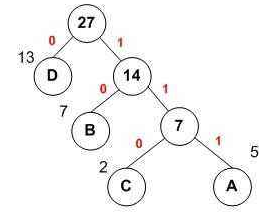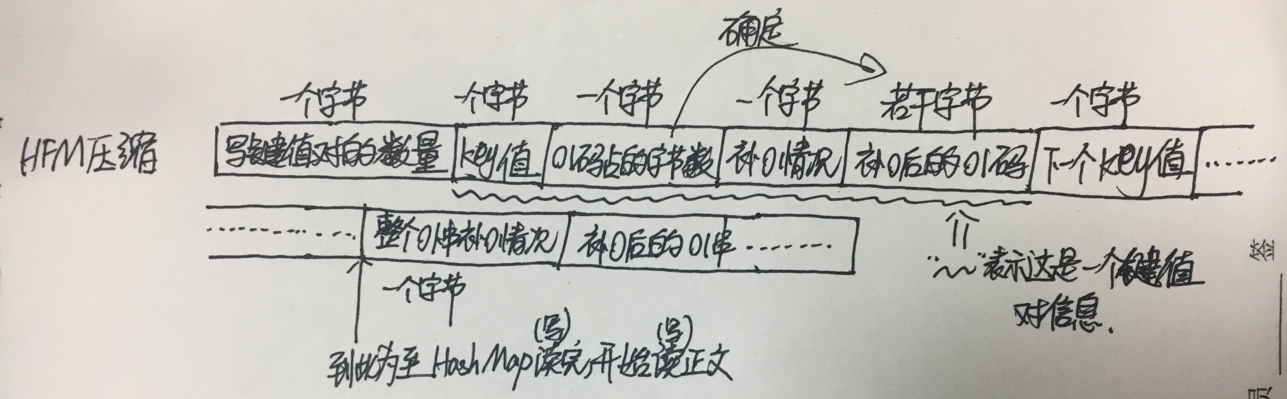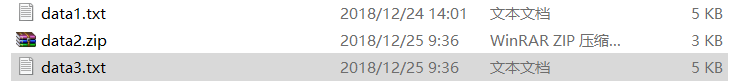• 实验内容：写出程序，利用哈弗曼编码实现对文件的压缩，并能解压文件。 实验步骤： 1、压缩 （1） 统计原始文件中各字节出现的概率（次数）； （2） 采用哈弗曼算法对各字节进行编码，建立哈弗曼对照表； a) 构造...
• 使用java实现的采用Huffman编码来实现文件的压缩解压，亲自测试，好使！
• 建立相应的huffman树 对上述字符进行编码 3 编码：根据编码表对输入的字符串进行编码压缩 并将编码后的字符串输出到compress txt文件中 4 译码：对compress txt中的压缩字符进行解压缩解压的答案输出到文件...压缩解压
• java编写的huffman编码对文本文件进行压缩解压，有完整的测试文件、java文件和测试结果文件，还附有详细的算法设计说明。良心资源，值得拥有！
• python版本为2.7.9，大家注意别下错了，里面有一个txt文件是进行压缩的，可以更改文件中的变量path1来对其他文件进行压缩解压，代码中有详细注释，实现过程虽然简单，但是包含自己很多一些独特的想法，自己的知识...
•Huffman
• jdk自带的ZipEntry类解压zip文件，中文文件会出现乱码，jar包是根据Apache的解压缩包进行改造的，也适合于Android使用
• 利用无失真信源编码方法中的哈夫曼编码进行程序设计实践，实现对文件的压缩解压操作。
•哈夫曼编码
• 哈夫曼编码的数据压缩解压，程序可运行，为完整的程序设计
• 4 译码：对compress txt中的压缩字符进行解压缩解压的答案输出到文件decompress txt文件中 5 比较decompress txt和input txt中的字符是否完全相同 并计算压缩压缩率 compress txt文件大小 input txt的文件大小...压缩解压
• 使用哈夫曼编码统计字符的频率作为权值来实现压缩技术 ，包括哈夫曼树的创建，构造哈夫曼编码，使用哈夫曼编码压缩文件，和解压文件
• 利用实现的最小堆实现霍夫曼编码，利用霍夫曼编码实现文件压缩解压。 包括最小堆，霍夫曼编码解压压缩四个部分，代码功能完善，对ASCII码英文文本有1.8压缩率。使用C++编写。（现只能压缩文本文件）
• 编码数据，实际的编码数据位数为dataBitCount，最后一个字节的数据如果是不满8位，余下的位用0作为padding 算法流程 压缩 读取图像文件，每个像素包含RGB三个色彩通道，每个通道占1个字节，这是编码的单元。 对读取...
• java端：返回类型非字符接口调用StringUtils#base64AndCompressJson进行编码压缩 ，返回类型为字符接口调用StringUtils#base64Andcompress js 端：引入压缩包中的js文件 ，调用deBase64AndUncompress进行解压解码base64
• 哈夫曼编码文件压缩解压 没整懂 这份代码竟然只能压缩文本文件，内容不能包含中文，不能解压大于 8 k 的 public static void main(String[] args) { // 只能压缩txt，压缩的文件不能有中文 String srcFile =...

哈夫曼编码文件压缩解压

没整懂
这份代码竟然只能压缩文本文件，而且内容不能包含中文，不能解压大于 8 k 的zip压缩文件

还有就是如果使用哈夫曼编码压缩的内容重复率不高，压缩的效果不明显，如果内容的重复率高压缩的效果好点public static void main(String[] args) {

// 只能压缩txt，压缩的文件不能有中文
String srcFile = "F:\\Temp\\test2.txt";
String dstFile = "F:\\Temp\\hufmanTxt.zip";
FileZip(srcFile, dstFile);

String srcFile2 = "F:\\Temp\\hufmanTxt.zip";
String dstFile2 = "F:\\Temp\\jieya.txt";
FileUnZip(srcFile2, dstFile2);

}

/**
*  调用封装
*/
public static byte[] hufmanZip(byte[] bytes){
List<hufNode> nodes = strToList(new String(bytes));
hufNode root = createHufmanTree(nodes);

getCodes(root, "", context);
byte[] zip = zip(bytes, codeMap);

return zip;
}

/**
*  哈夫曼编码 - 文件压缩
* @param srcFile
* @param dstFile
*/
public static void FileZip(String srcFile, String dstFile){
InputStream is = null;
ObjectOutputStream oos = null;
FileOutputStream os = null;

try {
// 输入流
is = new FileInputStream(srcFile);

// 创建 byte 数组接收输入流文件
byte[] b = new byte[is.available()];
// 读入 b 数组中

// 哈夫曼编码处理后的 字节数组
byte[] hufmanCodes = hufmanZip(b);

os = new FileOutputStream(dstFile);
// 输出流
oos = new ObjectOutputStream(os);

oos.writeObject(hufmanCodes);
// 把哈夫曼编码表也写入起来，文件恢复操作需要用到
oos.writeObject(codeMap);

System.out.println("压缩成功!! ");

} catch (Exception e) {
System.out.println(e.getMessage());
}finally {
try {
oos.close();
os.close();
is.close();
} catch (IOException e) {
e.printStackTrace();
}
}

}

/**
*  哈夫曼编码 -- 文件解压
* @param srcFile
* @param dstFile
*/
public static void FileUnZip(String srcFile, String dstFile){
InputStream is = null;
ObjectInputStream ois = null;
OutputStream os = null;
try {
is = new FileInputStream(srcFile);
ois = new ObjectInputStream(is);

// 哈夫曼编码处理后的字节数组
// 存入 b
Map<Byte, String> hufmanMap = (Map<Byte, String>) ois.readObject();

// 解析
byte[] decode = decode(hufmanMap, b);
os = new FileOutputStream(dstFile);
os.write(decode);

System.out.println("解压成功~~");

} catch (Exception e) {
System.out.println(e.getMessage());
} finally {
try {
os.close();
ois.close();
is.close();
} catch (IOException e) {
e.printStackTrace();
}
}
}展开全文zip java
• 运用哈夫曼编码压缩解压文件源代码，代码有详细的注释，很好的压缩解压的源代码
• 哈夫曼编码用于解压压缩的示例代码，非常简单易懂，C风格C++写法。
• 二维行程编码对图像压缩解压。利用线性四叉树的结构编写morton码和像素灰度值，然后存成一个线性表，就可以进行无损压缩
• 北京工业大学--算法作业3--huffman编码对文本文件进行压缩解压---Java代码 设计并实现贪心算法，利用huffman编码对文本文件进行压缩解压。 输入：一个文本文件 输出：压缩后的文件 算法过程： （1）统计文本...
• JPEG是用于灰度图与真彩图的静态图像压缩的国际标准，它采用的是以DCT（Discrete Cosine Transform，离散余弦变换）为基础的有损压缩算法。因为视频的帧内编码就是静态图像编码，所以JPEG的编码算法也用于MPEG视频...
• 利用实现的最小堆实现霍夫曼编码，利用霍夫曼编码实现文件压缩解压。 包括最小堆，霍夫曼编码解压压缩四个部分，代码功能完善，对ASCII码英文文本有1.8压缩率。使用C++编写。
• 哈夫曼编码实现文件的压缩和解压

万次阅读 多人点赞 2018-12-25 14:22:33
哈夫曼编码是基于哈夫曼树实现的一种文件压缩方式。 哈夫曼树：一种带权路径最短的最优二叉树，每个叶子结点都有它的权值，离根节点越近，权值越小（根节点权值为0，往下随深度增加以此类推），树的带权路径等于...

哈夫曼编码的概念

哈夫曼编码是基于哈夫曼树实现的一种文件压缩方式。
哈夫曼树：一种带权路径最短的最优二叉树，每个叶子结点都有它的权值，离根节点越近，权值越小（根节点权值为0，往下随深度增加依次加一），树的带权路径等于各个叶子结点的数值与其权值的乘积和。哈夫曼树如图：从图中我们可以看出，数据都存放在叶子结点中，且为了达到树的带权路径最短，我们把数值大的节点放在靠近根的位置，这棵树的带权路径长度为：23+53+72+131=48。接下来我们为每个节点赋予哈夫曼编码，假设从根节点出发，到左子树获得编码0，到右子树获得编码1，这样我们可以得到D的编码是0，B的编码是10，C的编码是110，A的编码是111。离根越近的节点对应的编码越短，节点的数值越大。那么，如何把哈夫曼编码应用在文档的压缩上呢？我们记文件中字符出现的次数为节点的数值，出现次数最多的字符会分配到哈夫曼树的靠近根节点的地方，自然也就会获得较短的哈夫曼编码。于是我们通过这种方式，使得文档中的字符获得不同的哈夫曼编码，因为出现频次高的字符对应编码较短，所以从文档中获取的字节被哈夫曼编码替换之后，会获使得其占用的总存储空间变小，实现压缩的效果。

实现哈夫曼压缩和解压的步骤详解

建立哈夫曼树：
1、使用IO流逐字节读取TXT文档。用一个数组（0~255,下标表示ASCII码）来保存不同字符出现的次数（对应位置加一）。
2、建一个节点类，保存节点对象的信息。将数组每一位表示的字符和出现频次存入创建的节点，把所有节点存入一个链表。
3、根据节点存储的频次值，对链表进行排序（从小到大）。
4、从链表中取出并删除最小的两个节点，创建一个他们的父节点，父节点不存字符，值为那两个节点的和，把那两个节点分别作为其左子节点和右子节点，最后把这个父节点存入链表。再次排序，取出并删除最小的两个节点，生成父节点，再存入…以此类推，最终生成一棵哈夫曼树。
5、对哈夫曼树进行遍历，使得叶子结点获得相应编码，同时把字符和它对应的哈夫曼编码存入HashMap。
哈夫曼压缩的实现：
1、再次读取原文档（之前第一次读取只是为了获取HashMap），根据HashMap中的字符与编码的键值对把整个文档转化为一串01码（此处可以用01字符串表示）。
2、准备将数据写入要压缩的目录。首先把HashMap写入（如果压缩文件中没有HashMap的信息，在解压的时候将无法还原）。HashMap包括两个部分，一部分是key值（即字符），占一个字节，另一部分是01字符串编码，若转为字节表示，可能小于8位有可能大于8位（即长度不确定），我们在写入时必须明确每个01串占据的字节个数，再者，因为我们是以字节的形式写数据，写数据的时候总位数应是8的整数倍，需要对01串末尾补0。我们具体是这样写HashMap的：写键值对的数量（占一个字节）；写key值（把字符转为ASCII值写入，占一个字节）；写01码占几个字节（是补0后的字节数，此信息占一个字节）；写补0情况（某位补0数，此处也占一个字节），写补零后的01码对应的若干字节。继续下一个键值对的写入…以此类推，直到整个HashMap的键值对都写完。
3、刚才写的是编码信息，接下来准备把整个原文档转换得到的01串写入，这也是我们之后需要还原的信息。刚才的流没有关闭，我们是继续写入的。因为这依然会遇到最后一个字节不足8位的情况，我们需要补0并记录补0情况。先写整个文档的补0情况（一个字节），再把补0后的01串以每8位为一个字节写入压缩文件。
压缩格式如图解压的实现：
1、先读取第一个字节，即编码个数，确定了我们需要读多少组数据。
2、开始正式读取键值对信息。读取key值，读取01码对应的字节数，读取补0情况，再读取表示01串的字节数据，去掉之前补的0，还原回0和1表示的字符串，即字符对应的哈夫曼编码，把读到的字符和哈夫曼编码保存在一个新建的HashMap中，需要注意的是此处key值存储为哈夫曼编码，value值存储为字符的信息。以此类推，直到读完所有键值对信息。
3、读整个文件补0个数，读取文件字节数据，去掉补的0，得到之前存入的哈夫曼编码01字符串。
4、确定希望解压的文件目录。逐位读取01字符串，将读到的位累加在一个临时字符串中，每读一位都拿这个临时字符串和HashMap进行对照，如果有对应key值，则获取对应字符信息写入流，把字符串置空，继续循环累加新的01串。最终读完后，解压目录中便得到了我们解压后的文件。

代码实现

1、节点类：

public class Node<T> implements Comparable<Node<T>>{
private T data;
private int weight;
private Node<T> left;
private Node<T> right;
public Node(T data,int weight)
{
this.data=data;
this.weight=weight;
}
/**
* 获取节点数据
*/
public String toString()
{
return "data:"+data+"   "+"weight:"+weight;
}
/**
* 节点权值比较方法
* @param o
* @return
*/
public int compareTo(Node<T> o) {
if(this.weight>o.weight)
return 1;
else if(this.weight<o.weight)
return -1;
return 0;
}
public void setData(T data)
{
this.data=data;
}
public void setWeight(int weight)
{
this.weight=weight;
}
public T getData()
{
return data;
}
public int getWeight()
{
return weight;
}
public void setLeft(Node<T> node)
{
this.left=node;
}
public void setRight(Node<T> node)
{
this.right=node;
}
public Node<T> getLeft()
{
return this.left;
}
public Node<T> getRight()
{
return this.right;
}
}

2、mian方法入口及建树的方法

public class HFMcompression {
public static void main(String[] args)
{
HFMcompression hc = new HFMcompression();
File file  = new File("E:\\workspace\\mayifan\\src\\com\\myf\\HFMcompression1223\\data1.txt");//源文件地址
FileOperation fo = new FileOperation();
int [] a = fo.getArrays(file);
System.out.println(Arrays.toString(a)); //打印
for(int i=0;i<list.size();i++)
{
System.out.println(list.get(i).toString());
}
Node<String> root = hc.CreateHFMTree(list); //建树
System.out.println("打印整棵树、、、、");
hc.inOrder(root); //打印整棵树
System.out.println("获取叶子结点哈夫曼编码");
HashMap<String,String> map = hc.getAllCode(root);//获取字符编码HashMap
String str = fo.GetStr(map, file);
System.out.println("转化得到的01字符串："+str);
File fileCompress = new File("E:\\workspace\\mayifan\\src\\com\\myf\\HFMcompression1223\\data2.zip");//压缩文件地址
fo.compressFile(fileCompress,map,str);  //生成压缩文件
File fileUncompress = new File("E:\\workspace\\mayifan\\src\\com\\myf\\HFMcompression1223\\data3.txt");//压缩文件地址
fo.uncompressFile(fileCompress,fileUncompress);//解压文件至fileUncompress处
}
/**
* 把获得的数组转化为节点并存在链表中
* @param arrays
* @return
*/
{
for(int i=0;i<arrays.length;i++)
{
if(arrays[i]!=0)
{
String ch = (char)i+"";
Node<String> node = new Node<String>(ch,arrays[i]); //构建节点并传入字符和权值
}
}
return list;
}
/**
* 对链表中的元素排序
* @param list
* @return
*/
{
for(int i=list.size();i>1;i--)
{
for(int j=0; j<i-1;j++)
{
Node<String> node1 = list.get(j);
Node<String> node2 = list.get(j+1);
if(node1.getWeight()>node2.getWeight())
{
int temp ;
temp = node2.getWeight();
node2.setWeight(node1.getWeight());
node1.setWeight(temp);
String tempChar;
tempChar = node2.getData();
node2.setData(node1.getData());
node1.setData(tempChar);
Node<String> tempNode = new Node<String>(null, 0);
tempNode.setLeft(node2.getLeft());
tempNode.setRight(node2.getRight());
node2.setLeft(node1.getLeft());
node2.setRight(node1.getRight());
node1.setLeft(tempNode.getLeft());
node1.setRight(tempNode.getRight());
}
}
}
}
/**
* 建树的方法
* @param list
*/
{
while(list.size()>1)
{
sortList(list); //排序节点链表
Node<String> nodeLeft = list.removeFirst();
Node<String> nodeRight = list.removeFirst();
Node<String> nodeParent = new Node<String>( null ,nodeLeft.getWeight()+nodeRight.getWeight());
nodeParent.setLeft(nodeLeft);
nodeParent.setRight(nodeRight);
}
System.out.println("根节点的权重："+list.get(0).getWeight());
return list.get(0);//返回根节点
}
public HashMap<String, String> getAllCode(Node<String> root)
{
HashMap<String, String> map = new HashMap<>();
inOrderGetCode("", map, root);
return map;
}
/**
* 查询指定字符的哈夫曼编码（中序遍历）
* @param code
* @param st
* @param root
* @return
*/
public void inOrderGetCode(String code ,HashMap<String, String> map,Node<String> root)
{
if(root!=null)
{
inOrderGetCode(code+"0",map,root.getLeft());
if(root.getLeft()==null&&root.getRight()==null)//存储叶子结点的哈夫曼编码
{
System.out.println(root.getData());
System.out.println(code);
map.put(root.getData(), code);
}
inOrderGetCode(code+"1",map,root.getRight());
}
}
/**
* 中序遍历输出整棵树
* @param root
* @return
*/
public void inOrder(Node<String> root)
{
if(root!=null)
{
inOrder(root.getLeft());
if(root.getData()!=null)
System.out.println(root.getData());
inOrder(root.getRight());
}
}
}

3、文件操作类（包括文件压缩对外的接口和文件解压对外的接口）：

public class FileOperation {
FileOutputStream fos;//申明文件输出流对象
FileInputStream fis; //申明文件写入流对象
/**
* 通过文件获取数组的方法
* @param str
*/
public int[] getArrays(File file)
{
int[] arrays = new int;
try{
FileInputStream fis = new FileInputStream(file);
int ascii=0;
{
arrays[ascii]++;
}
fis.close();
}catch(IOException e){
e.printStackTrace();
}
return arrays;
}
/**
* 读取文件获取01码
*/
public String GetStr(HashMap map,File file)
{
String str="";   //定义字符串储存01码
try{
FileInputStream fis = new FileInputStream(file);
int value=0;
{
str+=map.get((char)value+"");  //取单字符对应的01码，累加到字符串中
}
fis.close();
}catch(IOException e)
{
e.printStackTrace();
}
return str;
}
/**
* 写HashMap到文件（写入编码个数+第一个key+第一个value所占字节数+value最后一个字节的补0情况+第一个value的若干字节+下一个key+。。。。）
*/
public void writeHashMap(HashMap<String, String> map ,File file)
{
int size = map.size(); //获取编码的个数，即HashMap中的键值对个数
String temp=""; //存放临时8位01字符串
int value=0; //存放01字符串转化得到的ASCII值
try{
fos = new FileOutputStream(file);
fos.write(size);  //写HashMap长度
Set<String> keySet = map.keySet(); //获取HashMap存放key的容器
java.util.Iterator<String> it = keySet.iterator();//通过容器获取迭代器
while(it.hasNext()) //迭代判断，有下一个key
{
String key = it.next(); //取出下一个key
String code = map.get(key); //取出code
fos.write(key.charAt(0)); //写key值
int a = code.length()/8;//能存满的字节数
int b = code.length()%8;//剩余的位数
int c =1; //值对应的存储的字节数
if(b==0) //无剩余位
{
c=a;
fos.write(c);  //写code的字节数
fos.write(0);  //写补0数，为0个
for(int i=0;i<a;i++) //写code值
{
temp="";
for(int j=0;j<8;j++)
{
temp+=code.charAt(i*8+j);
}
value=StringToInt(temp);
fos.write(value); //逐一把code的每一位写出去
}
}
else
{
c=a+1;
fos.write(c); //写code的字节数
fos.write(8-b); //写补0数
for(int i=0;i<8-b;i++) //补0
{
code+="0";
}
for(int i=0;i<c;i++)
{
temp="";
for(int j=0;j<8;j++)
{
temp+=code.charAt(8*i+j);
}
value=StringToInt(temp);
fos.write(value); //逐一写code，包括补的0
}
}
}
}catch(IOException e){
e.printStackTrace();
}
}
/**
* 把文档转化为的HFM编码写入文件
*/
public void writeHFMcode(String HFMcode)
{
int len = HFMcode.length();  //获取HFMcode长度
int a = len/8;   //求出完整的字节的数目
int b = len%8;   //求出剩余的位数
String temp = ""; //临时存放8位数据
int value = 0; //存放8位01转化得到的值
try
{
if(b==0)  //无不足八位的部分，不需要补0
{
fos.write(0); //写补0数
for(int i=0;i<a;i++)
{
temp="";
for(int j=0;j<8;j++)
{
temp+=HFMcode.charAt(i*8+j);
}
value=StringToInt(temp);
fos.write(value); //写HFMcode
}
}
else   //需要补0
{
int c = 8-b; //计算补0数
fos.write(c); //写补0数
for(int i=0;i<c;i++) //补0
{
HFMcode+="0";
}
for(int i=0;i<a+1;i++)
{
temp="";
for(int j=0;j<8;j++)
{
temp+=HFMcode.charAt(i*8+j);
}
value=StringToInt(temp);
fos.write(value); //写HFMcode
}
}
fos.close(); //写完关闭资源
}
catch(IOException e)
{
e.printStackTrace();
}
}
/**
* 把01字符串转化为ASCII码
* @param temp
* @return
*/
public int StringToInt(String temp)
{
int value=0;
for(int i=0;i<8;i++)
{
int x = temp.charAt(i)-48;
if(x==1)    //为1则累加入value
{
value+=Math.pow(2,7-i);  //表示2的(7-i)次方
}
}
return value;
}
/**
* 把数值转化为01字符串
* @param value
*/
public String IntToString(int value)
{
String temp1=""; //存放反的字符串
String temp="";  //存放正的字符串
while(value>0) //逐渐取出各个二进制位数，字符串为反向的
{
temp1+=value%2;
value=value/2;
}
for(int i=temp1.length()-1;i>=0;i--)
{
temp+=temp1.charAt(i);
}
return temp;
}
/**
* 把数值转化为01字符串，数值范围在0~255,01串不超过8位
* @param value
*/
public String IntToStringEight(int value)
{
String temp1=""; //存放反的字符串
String temp="";  //存放正的字符串
while(value>0) //逐渐取出各个二进制位数，字符串为反向的
{
temp1+=value%2;
value=value/2;
}
{
temp1+="0";
}
for(int i=temp1.length()-1;i>=0;i--) //反向的字符串获取正向的字符串
{
temp+=temp1.charAt(i);
}
return temp;
}
/**
* 对外部的接口，实现把压缩后的数据和信息写入压缩文件
* @param fileCompress
*/
public void compressFile(File fileCompress,HashMap<String, String> map,String HFMcode)
{
writeHashMap(map, fileCompress);  //写HashMap的数据
writeHFMcode(HFMcode); //继续写HFMcode 01字符串
}
/**
* 解压获取HashMap
* @param fileCompress
*/
{
HashMap<String, String> mapGet = new HashMap<>();
try
{
fis=new FileInputStream(fileCompress);
String key = ""; //HashMap的键值对
String code= ""; //未去0的字符串
String codeRZ="";//去0的字符串
int length=0; //表示还原后的字符串的理论长度，解决字符串前面的0的问题
int byteNum=1; //当前code占了几个字节
int value=0; //临时储值
int zeroLength=0;//code没有1的时候的字符串长度
for(int i=0;i<keyNumber;i++)
{
{
for(int k=byteNum-1;k>=0;k--)
{
}
code=IntToString(value);//把数值转为01code
value=0;//清零
length=8*byteNum-code.length();//计算在前面要补多少0
if(code.length()==0)  //若code内数字都为0，只要去掉尾部即可
{
for(int k=0;k<zeroLength;k++)
{
codeRZ+="0";
}
}
else    //code值不为0，补充前面的0，去掉后面的0
{
for(int k=0;k<length;k++)
{
codeRZ+="0";
}
{
codeRZ+=code.charAt(k);
}
}
}
else  //有补0
{
for(int k=byteNum-1;k>=0;k--)
{
}
code=IntToString(value);//把数值转为01code
value=0;//清0
length=8*byteNum-code.length();//计算在前面要补多少0
if(code.length()==0)  //若code内数字都为0，只要去掉尾部即可
{
for(int k=0;k<zeroLength;k++)
{
codeRZ+="0";
}
}
else   //code值不为0，补充前面的0，去掉后面的0
{
for(int k=0;k<length;k++)
{
codeRZ+="0";
}
{
codeRZ+=code.charAt(k);
}
}
}
mapGet.put(codeRZ , key ); //把读取到的键值对存入创建的HashMap
codeRZ=""; //清空
}
}
catch(IOException e)
{
e.printStackTrace();
}
return mapGet;
}
/**
* 获取压缩文件中的数据，还原哈夫曼编码01串
*/
{
String str1=""; //存放获取到的直接的01字符串
String str=""; //存放去掉补0的字符串
int value=0;
String temp="";
try{
{
temp=IntToStringEight(value); //把每个字节的数据转化为八位的01
str1+=temp;
}
{
str+=str1.charAt(i)+"";
return str;
}
fis.close();
}
catch(IOException e)
{
e.printStackTrace();
}
return str1;
}
/**
* 写入文件的保存路径（写文件）
* @param str
* @param mapGet
* @param fileCompress
*/
public void writeFile(String str , HashMap<String, String> mapGet,File fileCompress)
{
try
{
fos = new FileOutputStream(fileCompress); //获取文件输出流
int len = str.length();//获取01串的长度
String temp=""; //临时存放段的01字符串
for(int i=0;i<len;i++)
{
temp+=str.charAt(i);
if(mapGet.containsKey(temp))
{
fos.write(mapGet.get(temp).charAt(0)); //一个字符的字符串转字符然后写出
temp="";
}
}
fos.close();
}
catch(IOException e)
{
e.printStackTrace();
}
}
/**
* 对外部的接口，实现解压文件，获取HashMap和文件内容
* @param fileCompress,压缩文件目录
* @param fileUncompress，解压到的目录
*/
public void uncompressFile(File fileCompress,File fileUncompress)
{
HashMap<String, String> mapGet = readHashMap(fileCompress); //获取哈希表
writeFile(str,mapGet,fileUncompress);  //写文件到保存路径
}
}

压缩、解压效果

1、压缩文件所占内存小于原文件，解压后的文件和原文件大小相同。如图data1是原文件，data2是压缩文件，data3的解压后的文件。我们可以发现压缩后的压缩包所占内存3KB<5KB。2、原文件和解压后的文件的内容展示：
data1.txt：data3.txt：解压后txt的信息和原文件完全一致。

展开全文哈夫曼编码 文件压缩 文件解压 哈夫曼树
• 主要介绍了Python实现压缩解压gzip大文件的方法,分析了Python针对压缩成gzip文件及解压gzip文件的方法,并给出了相应的封装类,需要的朋友可以参考下
• AVS（Audio Video coding Standard，音视频编码标准）是中国自主制订的数字电视、IPTV等音视频系统的基础性标准。AVS标准（GB/T 20090）包括系统、视频、音频、一致性测试、参考软件、数字版权管理、移动视频、用IP...AVS视频编码
• c语言版的数据结构课程设计----赫夫曼编码压缩解压文件，代码内容全是自己写的，绝无copy!
• 程序在Linux下能实现对任意文本文件的压缩解压，包括中文字符，英文字符等，最终压缩占空间大小比是 压缩文件：源文件约为1:2。...